# Dive into C++ Operators

22 Aug 2022
Intermediate
4.85K Views

In C++ language, certain symbols tell the compiler to perform specific mathematic and logical operations on the variables. These symbols are called “Operators”.

For example, consider this statement :

``` a = b + c;
```

In this statement, + is the operator and b & c are the operands. Therefore, in short, operators operate the operands.In this section, we will take a look at the different types of operators :

• Arithmetic operators

• Relational operators

• Logical operators

• Bitwise operators

• Assignment operators

• Misc. operators

## Arithmetic Operators

Arithmetic operators are used in C++ programming to perform mathematical operations on the operands.The list of arithmetic operators in C++ are as follows. Let’s assume we have two variables a = 16, b = 4.

Operator
Operation Performed
Operation
Description Example
+
a + b (16 + 4 = 20)
-
Subtraction
Subtracts the operands
a – b (16 – 4 = 12)
*
Multiplication
Multiplies the operands
a * b (16 * 4 = 64)
/
Division
Divides the numerator by denominator
a / b (16 / 4 = 4)
%
Modulus
Returns the reminder of the division
a % b (16 % 4 = 0)

#### Example Program

``` #include <iostream>

int main()
{
int a = 16;
int b = 4;
cout << "The sum of a and b is : " << a + b << '\n';
cout << "The difference of a and b is : " << a - b << '\n';
cout << "The product of a and b is : " << a * b << '\n';
cout << "The division of a and b is : " << a / b << '\n';
cout << "The modulus of a and b is : " << a % b << '\n';
}
```

The output of this program will be :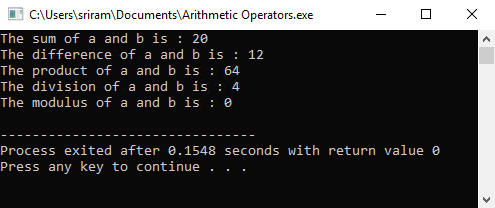### Types of Arithmetic Operators

There are two types of arithmetic operators :

#### Unary Operators

These operators work with only one operand. ++ (increment), -- (decrement) are the unary operators in C++ programming language.

Increment Operator : The ++ unary operator increments the value of an integer. The unary operator can be placed either before the operand or after the operand.

Example

++a –By adding the operator in front of the operand, the value is incremented by 1 immediately.

a++ -When the operator is behind the operand, the value is incremented by 1 only before the execution of the next statement.

Decrement Operator – The -- unary operator decrements the value of the integer. The decrement operator works the same way as the increment operator.

#### Binary Operators –

Binary operators work with two operands (+, -, *, /, %)

## Relational Operators

Relational operators compare the two operand values to see if they are either equal, greater than the other or lesser than the other.The list of relational operators in C++ are as follows. Let’s assume we have two variables a = 5, b = 10.

Operator
OperationPerformed
OperationDescription
Example
==
Equivalent
Checks if the two operands are equal or not
a == b (5 == 10); the condition is false
!=
Not Equivalent
Checks if the two operands are not the same a
!= b (5 != 10); the condition is true
>
Greater Than
Checks if the first operand is greater than second operand a
> b (5> 10); the condition is false
<
Lesser Than
Checks if the first operand is lesser than the second operand a
< b (5 < 10); the condition is true
>=
Greater Than or Equal
Checks if the first operand is greater than or equal to the second operand
a >= b (5 >= 10); the condition is false
<=
Lesser Than or Equal
Checks if the first operand is lesser than or equal to the second operand
a <= b (5 <= 10); the condition is true

#### Example Program

```#include <iostream>

int main()
{
int a = 5;
int b = 10;
cout << "Equivalent check : " << (a == b) << '\n';
cout << "Not Equivalent check : " << (a != b) << '\n';
cout << "Greater than check : " << (a > b) << '\n';
cout << "Lesser than check : " << (a < b) << '\n';
cout << "Greater than or equal to check : " << (a >= b) << '\n';
cout << "Lesser than or equal to check : " << (a <= b) << '\n';
}
```

The output of this program will be :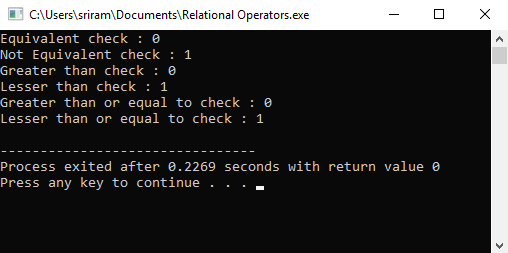## Logical Operators

Logical operators combine two or more different conditions and give the result.The list of logical operators in C++ are as follows. Let’s assume we have four variables a = 10, b = 5, c = 2, d = 4. The conditions are (a > b), (c==d)

Operator
Operation Performed
Operation Description
Example
&&
Logical AND
Checks if the two conditions are true. Only when both are true, the logical AND operator will return the value as true
(a > b) && (c == d) (10 > 5) && (2 == 4) True && False The condition is false
||
Logical OR
Checks if the two conditions are true. Even when one condition is true, the logical OR operator will return the value as true
(a > b) || (c == d) (10 > 5) || (2 == 4)True && False The condition is True
!
Logical NOT
Checks for the result of the two conditions. If the condition is true, the logical NOT operator will make it False. If the condition is false, the logical NOT operator will make it True.
(a > b) && (c == d) (10 > 5) && (2 == 4) True && False The condition is false The result will be True

#### Example Program

```#include <iostream>

int main()
{
int a = 10;
int b = 5;
int c = 2;
int d = 4;
cout << "Logical AND check : " << ((a > b) && (c == d)) << '\n';
cout << "Logical OR check : " << ((a > b) || (c == d)) << '\n';
cout << "Logical NOT check : " << !((a > b) && (c == d)) << '\n';
}
```

The output of this program will be :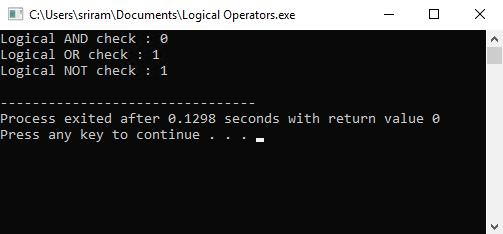To remember the logical operators easily, you can remember this table

Condition
Condition
AND Operator Result
OR Operator Result
True
True
True
True
True
False
False
True
True
False
False
True
False
True
False
True
False
False
False
False

## Bitwise Operators

The bitwise operators can be used to perform bit-level operations on the operands. The bit-level operations will be performed on the binary value of the variables.The list of bitwise operators in C++ are as follows. Let’s assume we have two variables a = 14, b = 4. The binary of 14 is 1110 and binary of 4 is 0100.

Operator
Operation Performed
Example
&
Binary AND
1110 0100 ------- 0100 – This is the binary for 4
|
Binary OR
1110
0100 ------- 1110 – This is the binary for 14
^
Binary XOR
1110 0100 ------- 1010 – This is the binary for 10
~
Binary One’s Complement
Changes the 0’s to 1’s and 1’s to 0s of the operand. This is a unary operator; hence it works only on one operand.E.g., the binary of 7 is 00001011. ~(00001011) is 11110100 which is 244.
<<
Binary Left Shift
This is a unary operator. For e.g., a = 7; the binary of 7 is 00001011. a << 2 means the operator will shift the bits towards left by 2. The new value will be 00101100 which is 44.
>>
Binary Right Shift
This is a unary operator. For e.g., a = 7; the binary of 7 is 00001011. a >>2 means the operator will shift the bits towards right by 2. The new value will be 00001011 which is 11.

#### Example Program

```#include<iostream>

int main()
{
int a = 14;
int b = 4;
cout << "Binary AND check : " << (a & b) << '\n';
cout << "Binary OR check : " << (a | b) << '\n';
cout << "Binary XOR check : " << (a ^ b) << '\n';
cout << "Binary One's Complement' check : " << ~a << '\n';
cout << "Binary Left Shift check : " << (a << 2) << '\n';
cout << "Binary Right Shift check : " << (a >> 2) << '\n';
}
```

The output of this program will be :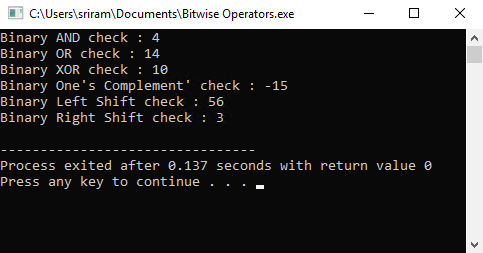## Assignment Operators

Assignment operators will assign the variable with values. As per the syntax, the left side of the operator is the variable and the right side of the operator is the value that is to be assigned to the variable. For example, x = 2; here

• x is the variable

• 2 is the value

• = is the assignment operator

Important note : The value on the right side of the operator should be the same as the data-type of the variable on the left. If the data type of the variable is integer (int), the value has to be an integer. If you provide the value as a character, the compiler will display an error when you compile the program.The list of assignment operators in C++ are as follows.

Operator
Operation Performed
Example
=
Equal
A + B = C. The sum of A and B will be assigned to C
+=
Adds the left variable value with the right variable value. Then assigns the result to the left variable.
A+=B. This is similar to writing A=A+B
-=
Subtracts the left variable value from right variable value. Then assigns the result to left variable.
A-=B. This is similar to writing A=A-B
*=
Multiplies the left variable value with the right variable value. Then assigns the result to the left variable.
A*=B. This is similar to writing A=A*B
/=
Divides the left variable value by the right variable value. Then assigns the result to the left variable.
A/=B. This is similar to writing A=A/B
%=
Performs the modulus of the left variable with right variable. Then assigns the result to the left variable.
A%=B. This is similar to writing A=A%B
<<=
Performs the left shift of the bits and assigns the result to the left variable.
A<<=2. This is similar to writing A=A<<2
>>=
Performs the right shift of the bits and assigns the result to the left variable.
A>>=2. This is similar to writing A=A>>2
&=
Performs the binary AND operation and assigns the result to the left variable.
A&=2. This is similar to writing A=A&2
|=
Performs the binary OR operation and assigns the result to the left variable.
A|=2. This is similar to writing A=A|2
^=
Performs the binary XOR operation and assigns the result to the left variable.
A^=2. This is similar to writing A=A^2

#### Example Program

```#include <iostream>

int main()
{
int a = 14;
int b = 4;
int c = a + b;
cout << "Equal to check : " << c << '\n';
cout << "A+=B check : " << (a += b) << '\n';
cout << "A-=B check : " << (a -= b) << '\n';
cout << "A*=B check : " << (a *= b) << '\n';
cout << "A/=B check : " << (a /= b) << '\n';
cout << "A%=B check : " << (a %= b) << '\n';
cout << "A<<=2 check : " << (a <<= 2) << '\n';
cout << "A>>=2 check : " << (a >>= 2) << '\n';
cout << "A&=2 check : " << (a &= 2) << '\n';
cout << "A|=2 check : " << (a |= 2) << '\n';
cout << "A^=2 check : " << (a ^= 2) << '\n';
}
```

The output of this program will be :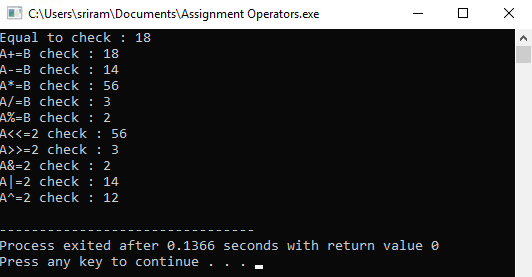## Miscellaneous Operators

In addition to the above operators, there are few miscellaneous operators in C++. The following are the list of misc. operators :

Operator
Operation Performed
Example
sizeofr
This unary operator returns the size of a variable. Sizeof can also be used to calculate the size of an array of elementsr
sizeof(char) will return 1sizeof(int) will return 4 For calculating the number of elements in the array, sizeof(arr)/size(arr
commar
This binary operator evaluates the first operand. Then it will discard the result and evaluate the second operand and returns the value.r
int a = (1 , 2). In this case, a is assigned the value of 2
conditionalr
The first expression will be executed. If the condition is true, the second expression will be executed.r
Otherwise (if false), the third expression will be executed. Expression_1 ? Expression_2 : Expression_3

#### Example Program

```#include <iostream>

int main()
{
int a = (1, 2);
int b[] = {1, 2, 3, 44, 55, 66, 777, 888, 999, 1234, 2345, 3456, 45678, 56789};
int x = 10;
int y = 15;
cout << "Size of Integer : " << sizeof(int) << '\n';
cout << "Size of Character : " << sizeof(char) << '\n';
cout << "Size of Double : " << sizeof(double) << '\n';
cout << "Size of Float : " << sizeof(float) << '\n';
cout << "Number of elements in Array is : " << sizeof(b)/sizeof(b) << '\n';
cout << "Value of variable a is : " << a << '\n';
x > y ? cout << "The value of x (10) is greater than y (15)" : cout << "The value of y (15) is greater than x (10)";
}
```

The output of this program will be :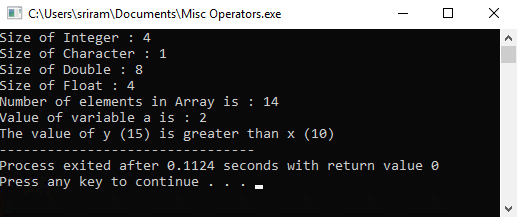##### Summary

In this section, we have covered the basics of the different operators in C++ with examples.

Learn to Crack Your Technical Interview